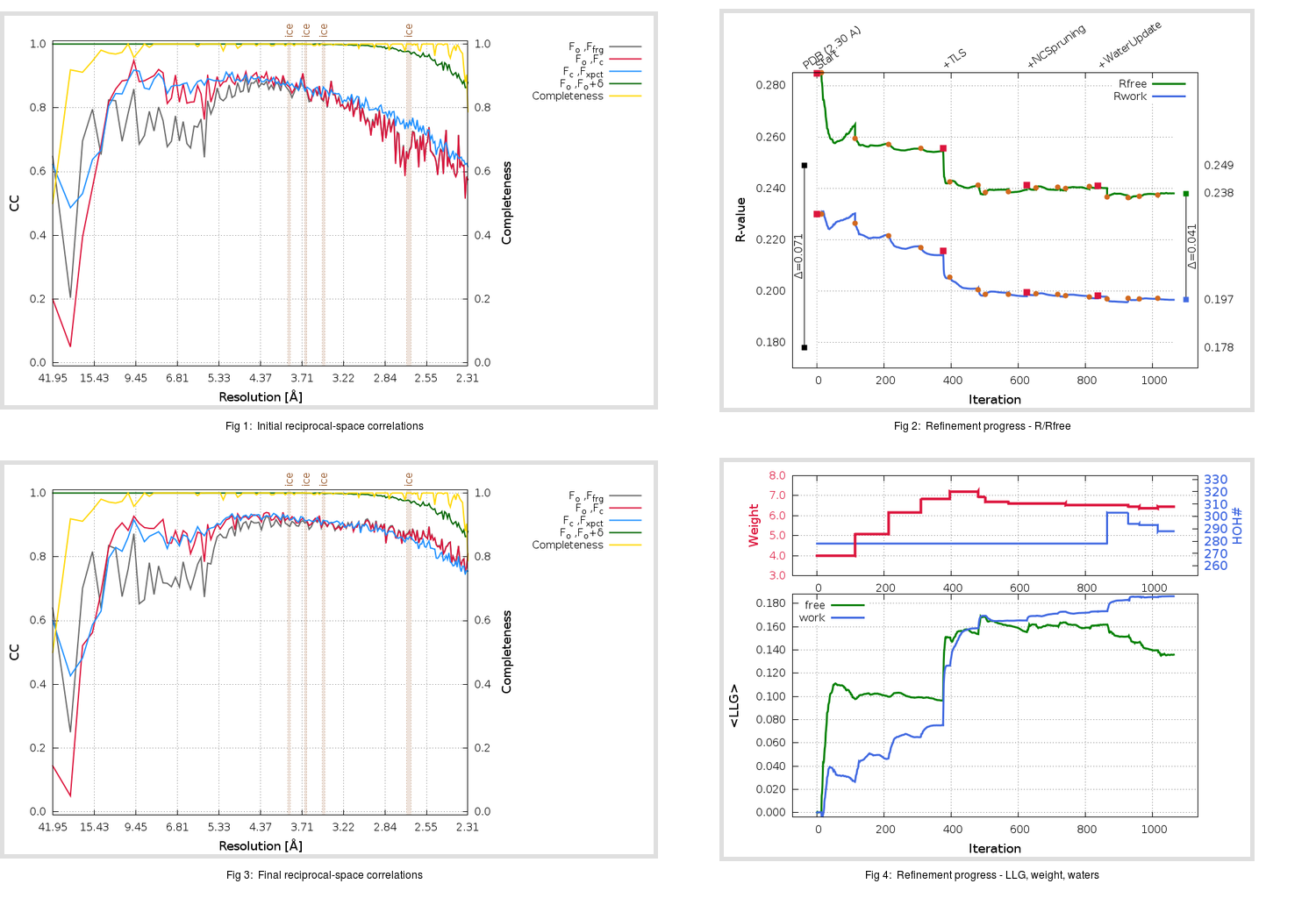Content:

```    Diffraction limits & principal axes of ellipsoid fitted to diffraction cut-off surface:
2.268         1.0000   0.0000   0.0000       a*
2.251         0.0000   1.0000   0.0000       b*
2.275         0.0000   0.0000   1.0000       c*
```

## Deposited

` `
 Date deposited Date data collection Resolution R, Rfree 20050630 20040825 2.30 0.1750 0.2490

Molprobity (CCP4 7.0 version) summary:

```Ramachandran outliers =   0.50 %
favored =  94.50 %
Rotamer outliers      =   9.76 %
C-beta deviations     =     8
Clashscore            =  15.29
RMS(bonds)            =   0.0207
RMS(angles)           =   1.92
MolProbity score      =   2.82
Resolution            =   2.30
R-work                =   0.1750
R-free                =   0.2490
```

```Number of waters      =   278

<B> (all atoms) =   47.51 ( sd =    9.45 ) for       5172 non-hydrogen atoms
<B>   (protein) =   46.64 ( sd =    8.75 ) for       4802 non-hydrogen atoms
<B>     (water) =   56.23 ( sd =    9.78 ) for        278 non-hydrogen atoms
<B>    (others) =   65.33 ( sd =    8.10 ) for         92 non-hydrogen atoms

B min/max       (all non-hydrogen atoms) =   22.03 /   82.04
B min/max   (protein non-hydrogen atoms) =   22.03 /   82.04
B min/max     (water non-hydrogen atoms) =   25.97 /   79.19
B min/max     (other non-hydrogen atoms) =   53.31 /   81.40
```

## BUSTER (re-)refinement

` `

Molprobity (CCP4 7.0 version) summary:

```Ramachandran outliers =   0.33 %
favored =  96.67 %
Rotamer outliers      =   5.71 %
C-beta deviations     =     3
Clashscore            =   5.34
RMS(bonds)            =   0.0115
RMS(angles)           =   1.61
MolProbity score      =   2.07
Resolution            =   2.30
R-work                =   0.1966
R-free                =   0.2381
```

```Number of waters      =   288

<B> (all atoms) =   55.52 ( sd =   17.51 ) for       5182 non-hydrogen atoms
<B>   (protein) =   54.85 ( sd =   17.35 ) for       4802 non-hydrogen atoms
<B>     (water) =   62.81 ( sd =   19.01 ) for        288 non-hydrogen atoms
<B>    (others) =   66.38 ( sd =    6.28 ) for         92 non-hydrogen atoms

B min/max       (all non-hydrogen atoms) =   24.36 /  300.00
B min/max   (protein non-hydrogen atoms) =   24.36 /  118.25
B min/max     (water non-hydrogen atoms) =   26.70 /  300.00
B min/max     (other non-hydrogen atoms) =   55.62 /   78.59
```

Refinement progression:Results:

` `
 File Remark 2A5K_aB_refine.01_04_refine.pdb.gz exact refinement commands are in header 2A5K_aB_refine.01_04_refine.mtz.gz including original deposited data and several re-refinement map coefficients 2A5K_aB_refine.01_04_BUSTER_model.cif.gz including any non-standard compound restraints 2A5K_aB_refine.01_04_BUSTER_refln.cif.gz